# (1 point) Estimate f(-3.01,-1.97) given that f(-3,-2) = 5, fx(-3,-2) = -2 and fy(-3, -2) =...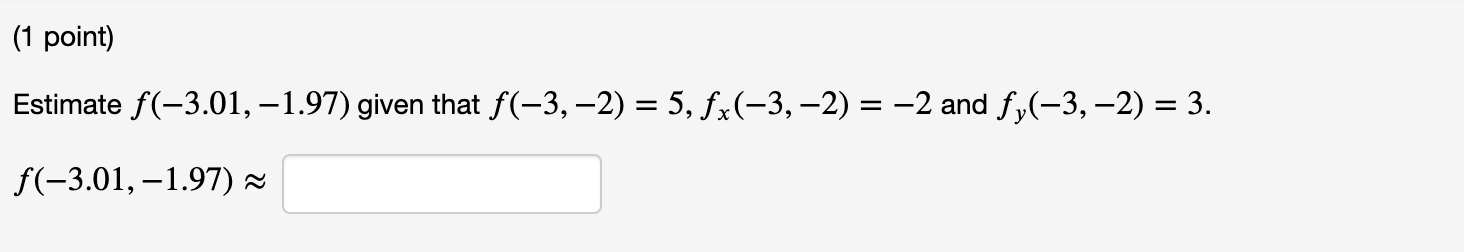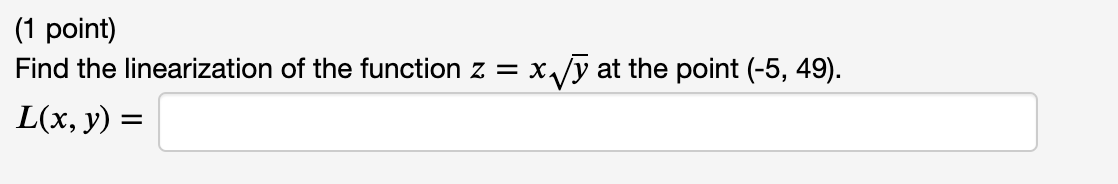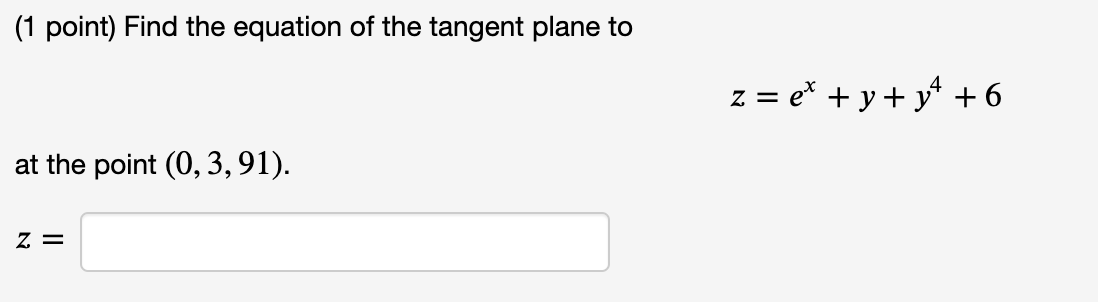(1 point) Estimate f(-3.01,-1.97) given that f(-3,-2) = 5, fx(-3,-2) = -2 and fy(-3, -2) = 3. f(-3.01,-1.97) ~
(1 point) Find the linearization of the function z = x,y at the point (-5, 49). L(x, y) =
(1 point) Find the equation of the tangent plane to z = ex + y + y4 + 6 at the point (0,3,91). z =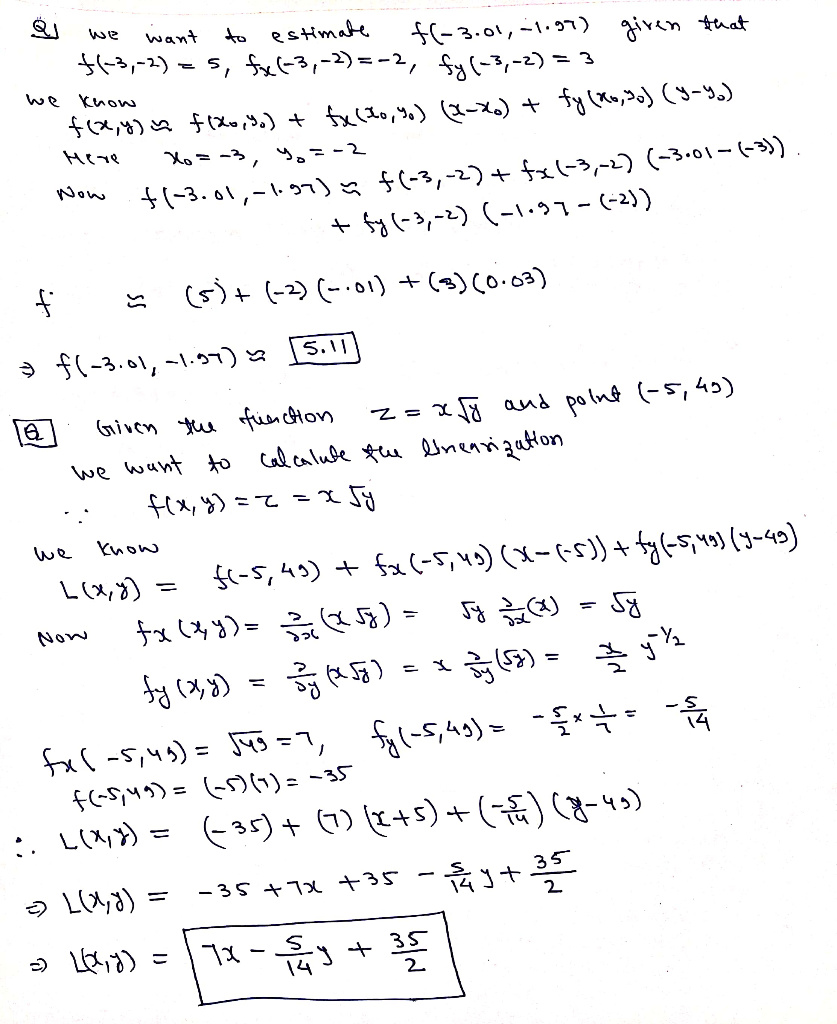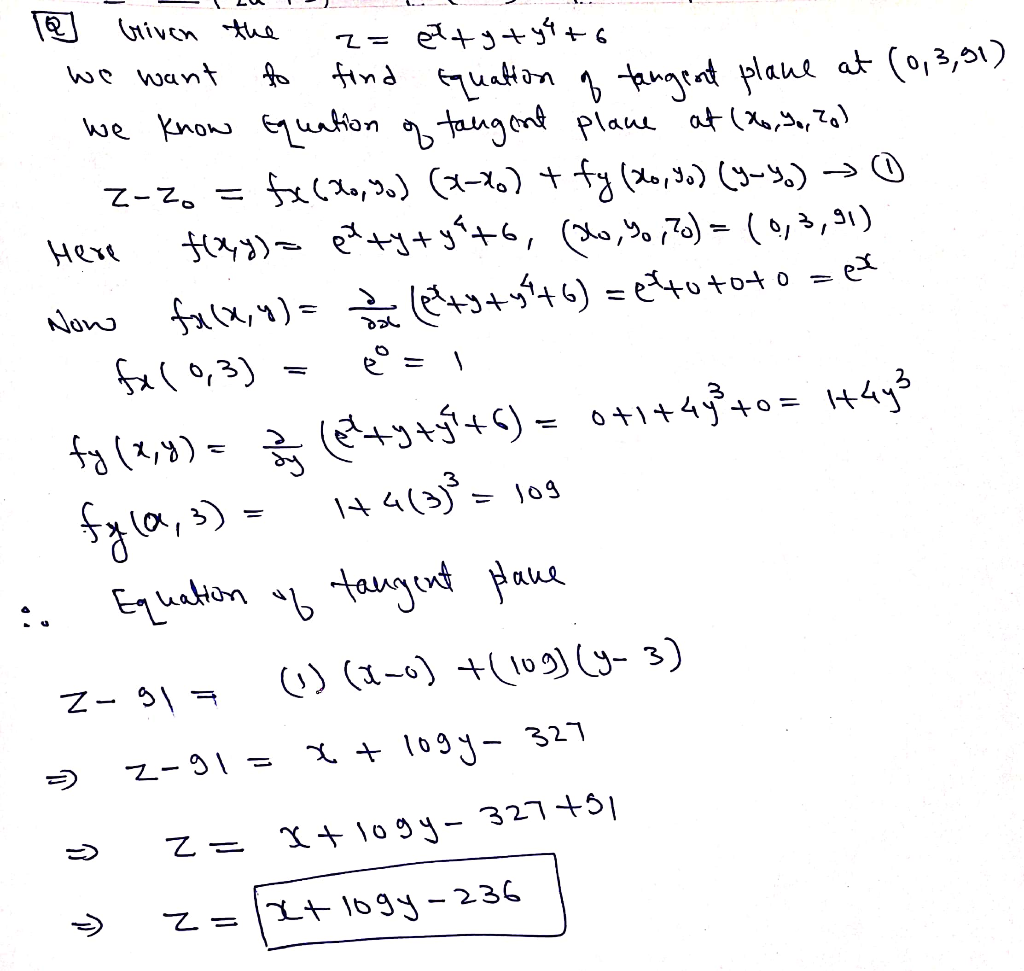#### Earn Coin

Coins can be redeemed for fabulous gifts.

Similar Homework Help Questions
• ### Summer-B3: Problem 7 Previous Problem Problem List Next Problem (1 point) Estimate f (2.02, 3.01) given...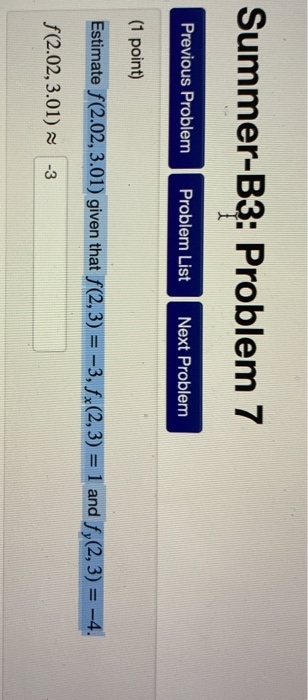Summer-B3: Problem 7 Previous Problem Problem List Next Problem (1 point) Estimate f (2.02, 3.01) given that f(2,3) = -3, fx(2, 3) = 1 and fy(2, 3) = -4. f(2.02,3.01) ~ -3

• ### 3. DO NOT USE CALCULATOR for this problem! Find the EXACT VALUES for all the parts. Given the function f(x,y) (a) Calculate the total differential of z at the point (x, y, z) (b) Use the total dif...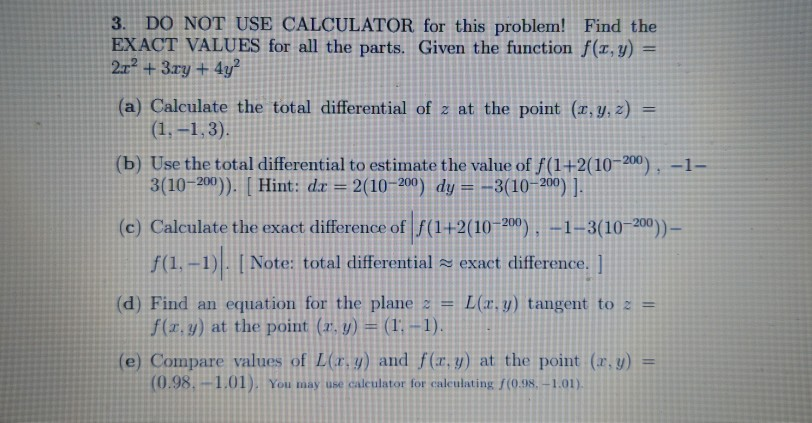3. DO NOT USE CALCULATOR for this problem! Find the EXACT VALUES for all the parts. Given the function f(x,y) (a) Calculate the total differential of z at the point (x, y, z) (b) Use the total differential to estimate the value of f(1+2(10200),-1 3(10-200). [ Hint : dz= 2(10-200) dy=_3(10-200)]. (c) Calculate the exact diffe ( f(1.-) I Note: total differentiala exact difference. ] rence of f(1+2(10-200)10 200))- (d) Find an equation for the plane s-L(x,y) tangent t(:-: f(z,y)...

• ### Check that the point (1,-1,2) lies on the given surface. Then, viewing the surface as a level surface for a function f(...

Check that the point (1,-1,2) lies on the given surface. Then, viewing the surface as a level surface for a function f(x,y,z), find a vector normal to the surface and an equation for the tangent plane to the surface at (1,-1,2). 2x^2-3y^2+z^2=3. Vector normal? and tangent plane?

• ### work. 1(a). Find fa, fy and fx for the function f(x, y, z) = xpez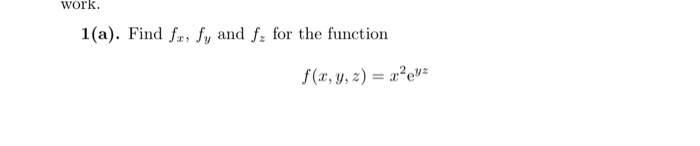work. 1(a). Find fa, fy and fx for the function f(x, y, z) = xpez

• ### (1 point) A stone is thrown from a rooftop at time t 0 seconds. Its position at time t (the components are measured in meters) is given by r()-бі-50+ (24.5-49:2) k. The origin is at the base of...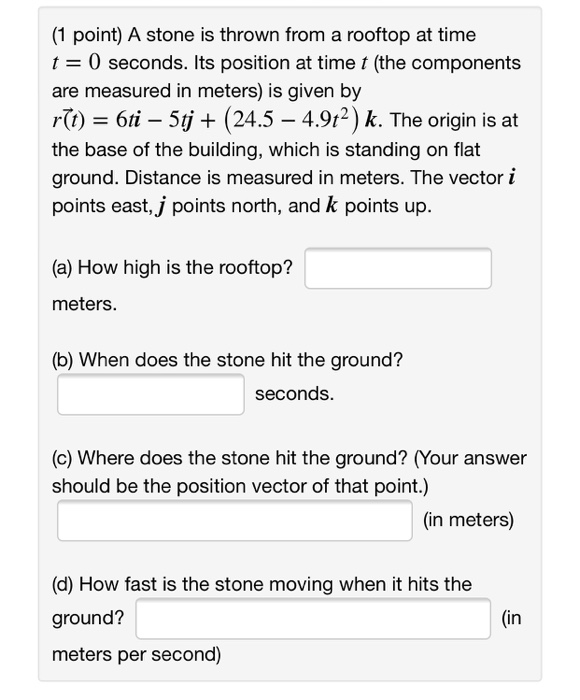(1 point) A stone is thrown from a rooftop at time t 0 seconds. Its position at time t (the components are measured in meters) is given by r()-бі-50+ (24.5-49:2) k. The origin is at the base of the bulding, which is standing on flat ground. Distance is measured in meters. The vector i points east,j points north, and k points up. (a) How high is the rooftop? meters. (b) When does the stone hit the ground? seconds (c) Where...

• ### 1. For the function f(x) e1+3x and the point P given by x 5 answer the...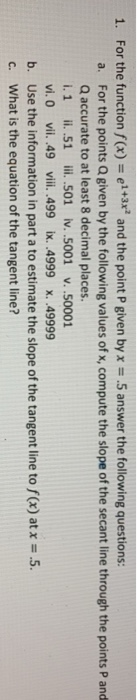1. For the function f(x) e1+3x and the point P given by x 5 answer the following questions: For the points Q given by the following values of x, compute the slope of the secant line through the points P and Q accurate to at least 8 decimal places. ii.51 l.501 iv. .5001 v. .50001 a. i. 1 vi. 0 vii. .49 vii. .499 ix. .4999 x. .49999 Use the information in part a to estimate the slope of the...

• ### Find fx, fy, and fz 5) f(x, y, z) = ln (xy)?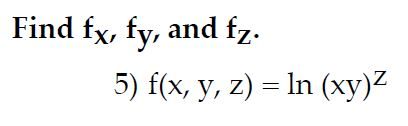Find fx, fy, and fz 5) f(x, y, z) = ln (xy)?

• ### Fx 0. Show that =-- dx Fy dy 8. Suppose y is a function of z, F(x, y) = 0, and F,メO. Show that d...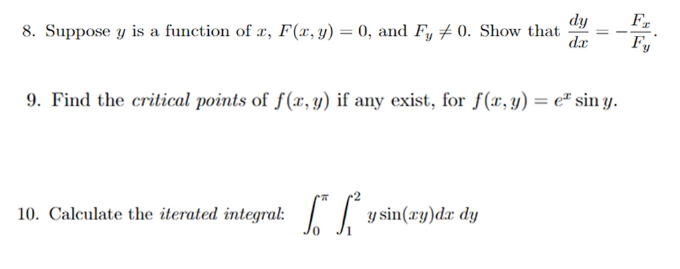Fx 0. Show that =-- dx Fy dy 8. Suppose y is a function of z, F(x, y) = 0, and F,メO. Show that dr--Fr 9. Fid the critical points of f(z, y) if any exist, for (a, y) = ex sin y 10. Calculate the iterated integral: ysin(zy)d dy Fx 0. Show that =-- dx Fy dy 8. Suppose y is a function of z, F(x, y) = 0, and F,メO. Show that dr--Fr 9. Fid the critical points...

• ### 1 Let f (z, y)5) Find the equation for the tangent plane to the graph of f at the point (3, 3) (Use symbolic notation a...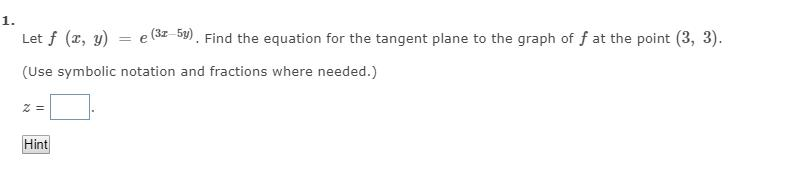1 Let f (z, y)5) Find the equation for the tangent plane to the graph of f at the point (3, 3) (Use symbolic notation and fractions where needed.) Hint 1 Let f (z, y)5) Find the equation for the tangent plane to the graph of f at the point (3, 3) (Use symbolic notation and fractions where needed.) Hint